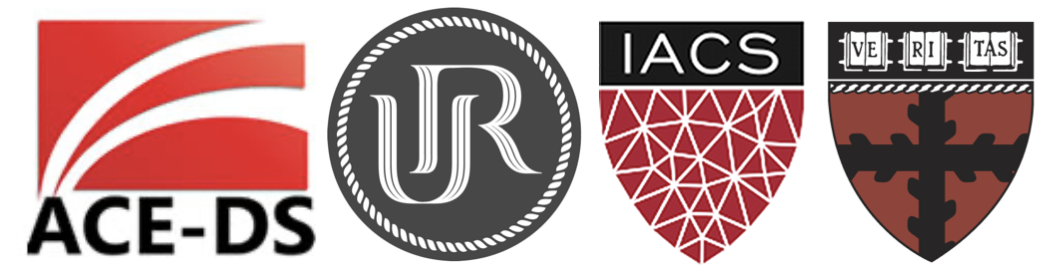# Machine Learning and Computation StatisticsThis is a teaching partnership between the Africa Center of Excellence in Data Science at the University of Rwanda and the Institute of Applied Computational Science at Harvard University.

View the Project on GitHub onefishy/Rwanda-Data-Science

# Week 0: Preparing for the Course

Students are expected to have fluency in `python` programming. You can familiarize yourself with `python` by completing online tutorials, for example: https://www.learnpython.org. In particular, students are expected to be able to manipulate data using `pandas` `DataFrames`, perform basic operations with `numpy` Arrays and make use of basic plotting functions (e.g. line chart, histogram, scatter plot, bar chart) from `matplotlib`:

1. `pandas` Basics
2. `numpy` Basics
3. `matplotlib` Basics For this course, we will be using Google Colab - a free cloud computing service that comes with pre-installed machine learning tools. Colab is built on Jupyter Notebooks, an open-source web application that allows you to create and share documents that contain live code, equations, visualizations and narrative text. You can familiarize yourself with the interface of Colab (and Jupyter) notebooks by reading the following tutorials (remember you don’t need to install anything!):

4. A Beginner’s Tutorial to Jupyter Notebooks
5. Introduction to Colab and Python

Finally, we assume that students have a strong foundation in (calculus and linear algebra based) statistics and probability. In particular, we will be working with random variables in this course and will be reasoning about them through their distributions. You should review these concepts before the course begins:

Course Preparation Exercise: Please review the following colab notebook:

Introduction to Python

Make sure you understand and are able to reproduce all basic operations demonstrated in the notebook. Complete the exercises in the notebook (the solutions to these exercises can be found in the notebook as well).